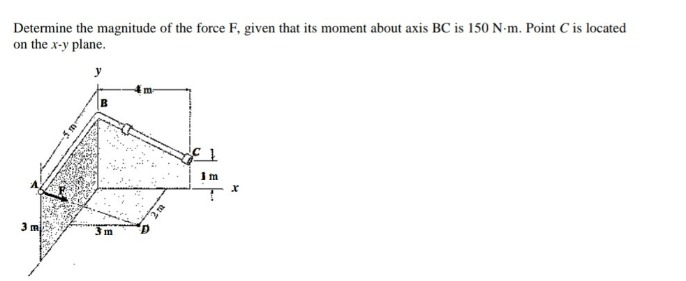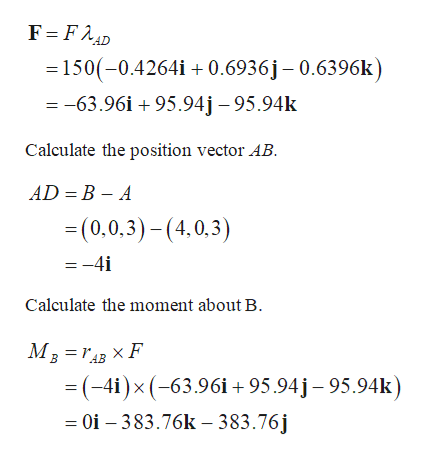# Determine the magnitude of the force F, given that its moment about axis BC is 150 N-m. Point C is locatedon the x-y planeymIm3 mm

Question
56 views

determine the magnitude of the force f given that its moment about the axis bc is 150 n·mhelp_outlineImage TranscriptioncloseDetermine the magnitude of the force F, given that its moment about axis BC is 150 N-m. Point C is located on the x-y plane y m Im 3 m m fullscreen
check_circle

Step 1

Note down the coordinates:

Step 2

Calculate the force vector...help_outlineImage TranscriptioncloseF FAD =150(-0.4264i +0.6936j- 0.6396k) =-63.96i +95.94j - 95.94k Calculate the position vector AB AD B - A (0,0,3)-(4,0,3) =-4i Calculate the moment about B MBr F =(-4i)x (-63.96i+95.94j- 95.94k) = Oi - 383.76k - 383.76j fullscreen

### Want to see the full answer?

See Solution

#### Want to see this answer and more?

Solutions are written by subject experts who are available 24/7. Questions are typically answered within 1 hour.*

See Solution
*Response times may vary by subject and question.
Tagged in

### Mechanical Engineering